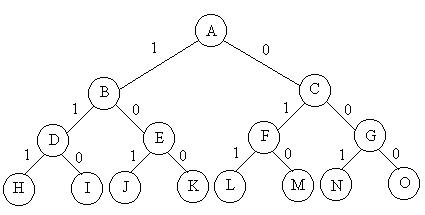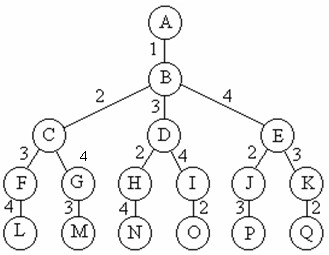# 1、概念

回溯算法实际上一个类似枚举的搜索尝试过程，主要是在搜索尝试过程中寻找问题的解，当发现已不满足求解条件时，就“回溯”返回，尝试别的路径。

回溯法是一种选优搜索法，按选优条件向前搜索，以达到目标。但当探索到某一步时，发现原先选择并不优或达不到目标，就退回一步重新选择，这种走不通就退回再走的技术为回溯法，而满足回溯条件的某个状态的点称为“回溯点”。

许多复杂的，规模较大的问题都可以使用回溯法，有“通用解题方法”的美称。

# 2、基本思想

在包含问题的所有解的解空间树中，按照深度优先搜索的策略，从根结点出发深度探索解空间树。当探索到某一结点时，要先判断该结点是否包含问题的解，如果包含，就从该结点出发继续探索下去，如果该结点不包含问题的解，则逐层向其祖先结点回溯。（其实回溯法就是对隐式图的深度优先搜索算法）。

若用回溯法求问题的所有解时，要回溯到根，且根结点的所有可行的子树都要已被搜索遍才结束。

而若使用回溯法求任一个解时，只要搜索到问题的一个解就可以结束。

# 3、用回溯法解题的一般步骤：

（1）针对所给问题，确定问题的解空间：

首先应明确定义问题的解空间，问题的解空间应至少包含问题的一个（最优）解。

（2）确定结点的扩展搜索规则

（3）以深度优先方式搜索解空间，并在搜索过程中用剪枝函数避免无效搜索。

# 4、算法框架

（1）问题框架

设问题的解是一个n维向量(a1,a2,………,an),约束条件是ai(i=1,2,3,…..,n)之间满足某种条件，记为f(ai)。

（2）非递归回溯框架

   1: int a[n],i;
   2: 初始化数组a[];
   3: i = 1;
   4: while (i>0(有路可走)   and  (未达到目标))  // 还未回溯到头
   5: {
   6:     if(i > n)                                              // 搜索到叶结点
   7:     {
   8:           搜索到一个解，输出；
   9:     }
  10:     else                                                   // 处理第i个元素
  11:     {
  12:           a[i]第一个可能的值；
  13:           while(a[i]在不满足约束条件且在搜索空间内)
  14:           {
  15:               a[i]下一个可能的值；
  16:           }
  17:           if(a[i]在搜索空间内)
  18:          {
  19:               标识占用的资源；
  20:               i = i+1;                              // 扩展下一个结点
  21:          }
  22:          else
  23:         {
  24:               清理所占的状态空间；            // 回溯
  25:               i = i –1;
  26:          }
  27: }

（3）递归的算法框架

回溯法是对解空间的深度优先搜索，在一般情况下使用递归函数来实现回溯法比较简单，其中i为搜索的深度，框架如下：

 int a[n];
   2: try(int i)
   3: {
   4:     if(i>n)
   5:        输出结果;
   6:      else
   7:     {
   8:        for(j = 下界; j <= 上界; j=j+1)  // 枚举i所有可能的路径
   9:        {
  10:            if(fun(j))                 // 满足限界函数和约束条件
  11:              {
  12:                 a[i] = j;
  13:               ...                         // 其他操作
  14:                 try(i+1);
  15:               回溯前的清理工作（如a[i]置空值等）;
  16:               }
  17:          }
  18:      }
  19: }

  回溯法有“通用解题法”之称。用它可以系统地搜索问题的所有解。回溯法是一个既带有系统性又带有跳跃性的搜索算法。    在包含问题的所有解的解空间树中，按照深度优先搜索的策略，从根结点出发深度探索解空间树。当探索到某一结点时，要先判断该结点是否包含问题的解，如果包含，就从该结点出发继续探索下去，如果该结点不包含问题的解，则逐层向其祖先结点回溯。（其实回溯法就是对隐式图的深度优先搜索算法）。若用回溯法求问题的所有解时，要回溯到根，且根结点的所有可行的子树都要已被搜索遍才结束。 而若使用回溯法求任一个解时，只要搜索到问题的一个解就可以结束。 1.回溯法的解题步骤

(1)针对所给问题，定义问题的解空间；
(2)确定易于搜索的解空间结构；
(3)以深度优先方式搜索解空间，并在搜索过程中用剪枝函数避免无效搜索。
2.子集树与排列树下面的两棵解空间树是回溯法解题时常遇到的两类典型的解空间树。(1)当所给问题是从n个元素的集合S中找出S满足某种性质的子集时，相应的解空间树称为子集树。例如从n个物品的0-1背包问题(如下图)所相应的解空间树是一棵子集树，这类子集树通常有2^n个叶结点，其结点总个数为2^(n+1)-1。遍历子集树的算法需Ω(2^n)计算时间。(2)当所给问题是确定n个元素满足某种性质的排列时，相应的解空间树称为排列树。例如旅行售货员问题(如下图)的解空间树是一棵排列树，这类排列树通常有n!个叶结点。遍历子集树的算法需Ω(n!)计算时间。[java]
view plain
copy

/**  * output(x)     记录或输出得到的可行解x  * constraint(t) 当前结点的约束函数  * bount(t)      当前结点的限界函数  * @param t  t为当前解空间的层数  */  void backtrack(int t){      if(t >= n)          output(x);      else          for (int i = 0; i <= 1; i++) {              x[t] = i;              if(constraint(t) && bount(t))                  backtrack(t+1);          }  }
用回溯法搜索排列树的一般算法可描述为：

[java]
view plain
copy

/**  * output(x)     记录或输出得到的可行解x  * constraint(t) 当前结点的约束函数  * bount(t)      当前结点的限界函数  * @param t  t为当前解空间的层数  */  void backtrack(int t){      if(t >= n)          output(x);      else          for (int i = t; i <= n; i++) {              swap(x[t], x[i]);              if(constraint(t) && bount(t))                  backtrack(t+1);              swap(x[t], x[i]);          }  }
3.回溯法的应用例子(a)子集树（为了便于描述算法，下列方法使用了较多的全局变量）

I.输出集合S中所有的子集，即limit为all；
II.输出集合S中限定元素数量的子集，即limit为num；
III.输出集合S中元素奇偶性相同的子集，即limit为sp。

[java]
view plain
copy

public class Subset {                private static int[] s = {1,2,3,4,5,6,7,8};      private static int n = s.length;      private static int[] x = new int[n];            /**      * 输出集合的子集      * @param limit  决定选出特定条件的子集      * 注：all为所有子集,num为限定元素数量的子集,      *    sp为限定元素奇偶性相同，且和小于8。      */      public static void all_subset(String limit){          switch(limit){          case "all":backtrack(0);break;          case "num":backtrack1(0);break;          case "sp":backtrack2(0);break;          }      }              /**      * 回溯法求集合的所有子集，依次递归      * 注：是否回溯的条件为精髓      * @param t      */      private static void backtrack(int t){          if(t >= n)              output(x);          else              for (int i = 0; i <= 1; i++) {                  x[t] = i;                  backtrack(t+1);              }                }            /**      * 回溯法求集合的所有(元素个数小于4)的子集，依次递归      * @param t      */      private static void backtrack1(int t){          if(t >= n)              output(x);          else              for (int i = 0; i <= 1; i++) {                  x[t] = i;                  if(count(x, t) < 4)                      backtrack1(t+1);              }                }        /**      * (剪枝)      * 限制条件：子集元素小于4,判断0~t之间已被选中的元素个数，      *        因为此时t之后的元素还未被递归,即决定之后的元素      *        是否应该被递归调用      * @param x      * @param t      * @return      */      private static int count(int[] x, int t) {          int num = 0;          for (int i = 0; i <= t; i++) {              if(x[i] == 1){                  num++;              }          }          return num;      }        /**      * 回溯法求集合中元素奇偶性相同，且和小于8的子集,依次递归      * @param t      */      private static void backtrack2(int t){          if(t >= n)              output(x);          else              for (int i = 0; i <= 1; i++) {                  x[t] = i;                  if(legal(x, t))                      backtrack2(t+1);              }                }            /**      * 对子集中元素奇偶性进行判断，还需元素的数组和小于8      * @param x      * @param t      * @return      */      private static boolean legal(int[] x, int t) {          boolean bRet = true;   //判断是否需要剪枝          int part = 0;  //奇偶性判断的基准                    for (int i = 0; i <= t; i++) {  //选择第一个元素作为奇偶性判断的基准              if(x[i] == 1){                  part = i;                  break;              }          }                    for (int i = 0; i <= t; i++) {              if(x[i] == 1){                  bRet &= ((s[part] - s[i]) % 2 == 0);              }                            }            int sum = 0;          for(int i = 0; i <= t; i++){              if(x[i] == 1)                  sum += s[i];          }          bRet &= (sum < 8);                        return bRet;      }          /**      * 子集输出函数      * @param x      */      private static void output(int[] x) {          for (int i = 0; i < x.length; i++) {              if(x[i] == 1){                  System.out.print(s[i]);              }          }          System.out.println();         }    }

(b) 排列树（为了便于描述算法，下列方法使用了较多的全局变量）

I.输出集合S中所有的排列，即limit为all；
II.输出集合S中元素奇偶性相间的排列，即limit为sp。

[java]
view plain
copy

public class Permutation {        private static int[] s = {1,2,3,4,5,6,7,8};      private static int n = s.length;      private static int[] x = new int[n];            /**      * 输出集合的排列      * @param limit  决定选出特定条件的子集      * 注：all为所有排列,sp为限定元素奇偶性相间。      */      public static void all_permutation(String limit){          switch(limit){          case "all":backtrack(0);break;          case "sp":backtrack1(0);break;          }      }              /**      * 回溯法求集合的所有排列，依次递归      * 注：是否回溯的条件为精髓      * @param t      */      private static void backtrack(int t){          if(t >= n)              output(s);          else              for (int i = t; i < n; i++) {  //没看懂没看懂                swap(i, t, s);                  backtrack(t+1);                  swap(i, t, s);              }                }        /**      * 回溯法求集合中元素奇偶性相间的排列,依次递归      * @param t      */      private static void backtrack1(int t){          if(t >= n)              output(s);          else              for (int i = t; i < n; i++) {                  swap(i, t, s);                  if(legal(x, t))                      backtrack1(t+1);                  swap(i, t, s);              }                }            /**      * 对子集中元素奇偶性进行判断      * @param x      * @param t      * @return      */      private static boolean legal(int[] x, int t) {          boolean bRet = true;   //判断是否需要剪枝                    //奇偶相间,即每隔一个数判断奇偶相同          for (int i = 0; i < t - 2; i++) {              bRet &= ((s[i+2] - s[i]) % 2 == 0);          }                        return bRet;      }          /**      * 元素交换      * @param i      * @param j      */      private static void swap(int i, int j,int[] s) {          int tmp = s[i];          s[i] = s[j];          s[j] = tmp;      }            /**      * 子集输出函数      * @param x      */      private static void output(int[] s) {          for (int i = 0; i < s.length; i++) {                  System.out.print(s[i]);          }          System.out.println();         }  }